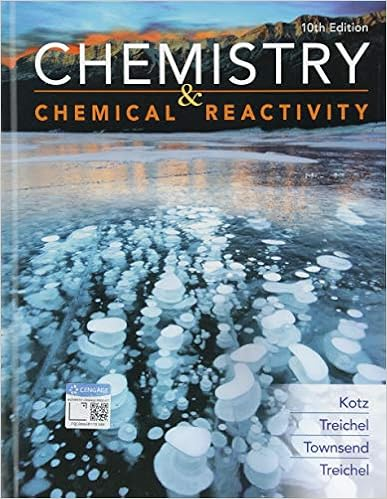# What was the expected volume of hydrogen produced in

• Test Prep
• 2
• 96% (193) 185 out of 193 people found this document helpful

This preview shows page 1 - 2 out of 2 pages.

##### We have textbook solutions for you!
The document you are viewing contains questions related to this textbook.The document you are viewing contains questions related to this textbook.
Chapter 4 / Exercise 10
Chemistry & Chemical Reactivity
Kotz/TreichelExpert Verified
What was the expected volume of hydrogen produced in the reaction in part 2? Compare your result with the value youmeasured in the lab and calculate the percent error using the formula below. 2.
Compare the experimental value for the molar volume at 21.5 ºC obtained in part 2 with the value listed in the Backgroundsection of the lab manual. The molar mass of Zn is 65.38 g/mol. Calculate the experimental error according to the equationbelow.3.
1 of 23/11/2018, 8:57 PM
##### We have textbook solutions for you!
The document you are viewing contains questions related to this textbook.The document you are viewing contains questions related to this textbook.
Chapter 4 / Exercise 10
Chemistry & Chemical Reactivity
Kotz/TreichelExpert Verified
Calculate and compare the value for the molar volume at 21.5 °C obtained in part 2 with the value for the molar volume at 21.5°C obtained in part 1. The molar mass of Zn is 65.38 g/mol.4.
How did using twice the amount of Zn in the second part of the experiment impact the molar volume? Explain your answer.5.
From the data recorded in the table below, calculate the pressure that would be observed.6.
2 of 23/11/2018, 8:57 PM
•••